# Cats

Two cats caught two mice in two days. How many mouses will catch 6 cats for 6 days?

Correct result:

x =  18

#### Solution: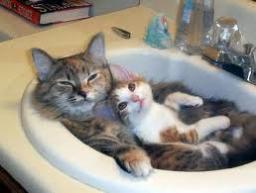Our examples were largely sent or created by pupils and students themselves. Therefore, we would be pleased if you could send us any errors you found, spelling mistakes, or rephasing the example. Thank you!

Please write to us with your comment on the math problem or ask something. Thank you for helping each other - students, teachers, parents, and problem authors.Tips to related online calculators
Check out our ratio calculator.

## Next similar math problems:

• Wooden blockA cuboid-shaped wooden block has 6 cm length, 4 cm breadth, and 1 cm height. Two faces measuring 4 cm x 1 cm are colored in black. Two faces measuring 6 cm x 1 cm are colored in red. Two faces measuring 6 cm x 4 cm are colored in green. The block is divid
• Wax candles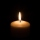A company makes wax candles in the shape of a cylinder. Each candle has a diameter of 4 inches and a height of 7 inches. If the company used 7561.12 in3 of wax, how many candles did it make? Use 3.14, and do not round your answer
• Positive integersSeveral positive integers are written on the paper. Michaella only remembered that each number was half the sum of all the other numbers. How many numbers could be written on paper?
• DozenWhat is the product of 26 and 5? Write the answer in Arabic numeral. Add up the digits. How many of this is in a dozen? Divide #114 by this
• Quarantine cupcakes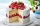Mr. Honse was baking quarantine cupcakes. Mrs. Carr made twice as many as Mr. Honse. Ms. Sanchez made 12 cupcakes more than Mr. Honse. If they put all their cupcakes together (which they can’t because. .. quarantine!) they would have 108 cupcakes. How may
• Job applicantsJob applicants: three-fourths of applicants had experience for a position. The number that did not have prior experience was 36. How many people applied for the job?
• Celsius degreesThe temperature on Monday was 5 celsius. The temperature on Thursday was 7 degrees less than the temperature on Monday. What was the temperature on Thursday?
• The temperature 8The temperature on Monday was –6°C. On Tuesday the temperature was 3 degrees lower. Write down the temperature on Tuesday.
• The outdoorThe outdoor temperature was 60 degrees Fahrenheit at midnight. The temperature went down 3 degrees each hour for the next 4 hours. What was the temperature at 4 A. M. ?
• Evaluate expressionIf x=2, y=-5 and z=3 what is the value of x-2y
• Temperature 13Work out the temperature after each change. The temperature starts at 6°C and falls by 13°C. The temperature starts at 2°C and falls by 8°C
• Evaluate 5Evaluate expression x2−7x+12x−4 when x=−1
• Evaluate - order of opsEvaluate the expression: 32+2[5×(24-6)]-48÷24 Pay attention to the order of operation including integersIn about 12 hours in North Dakota the temperature rose from -33 degrees farenheit to 50 degrees farenheit. By how much did the temperature change?Evaluate expression: (-1)2 . 12 – 6 : 3 + (-3) . (-2) + 22 – (-3) . 2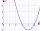Two integers, a and b, have a product of 36. What is the least possible sum of a and b?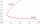The difference of two numbers is 1375. If their exact quotient is 12. Find the two numbers